Question

# 4. Consider a unity-feedback control system with the following open-loop transfer function: G(s)3 Sketch a Nyquist...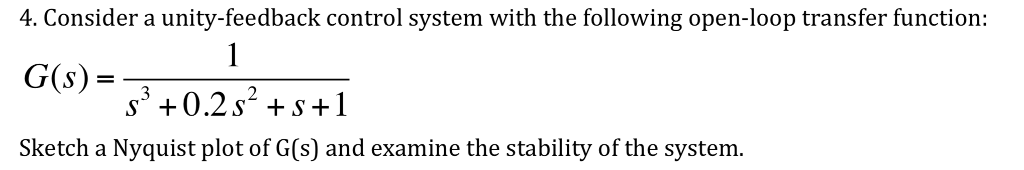4. Consider a unity-feedback control system with the following open-loop transfer function: G(s)3 Sketch a Nyquist plot of G(s) and examine the stability of the system.

We need at least 9 more requests to produce the answer.

1 / 10 have requested this problem solution

The more requests, the faster the answer.

All students who have requested the answer will be notified once they are available.

#### Earn Coins

Coins can be redeemed for fabulous gifts.

Similar Homework Help Questions
• ### Consider a unity-feedback control system with the following open-loop transfer G(s)

Consider a unity-feedback control system with the following open-loop transfer G(s) = 1/(s3+0.2s2 +s+1) Draw a Nyquist plot of G(s). You may draw the simple Nyquist plot

• ### 2. A unity feedback system has the following open-loop transfer function -0.5s + 0.5 G(s)i a)...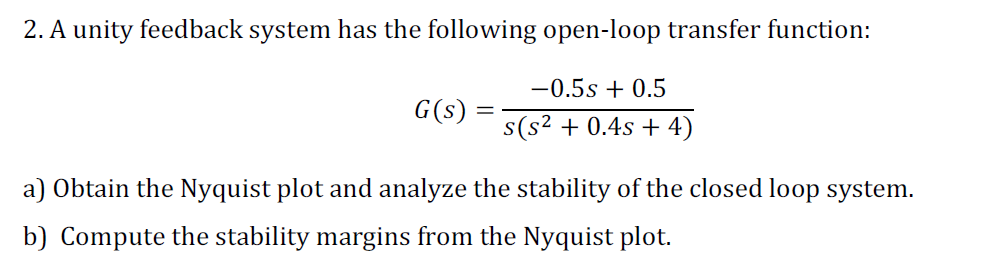2. A unity feedback system has the following open-loop transfer function -0.5s + 0.5 G(s)i a) Obtain the Nyquist plot and analyze the stability of the closed loop system b) Compute the stability margins from the Nyquist plot.

• ### A unity feedback control system has the following open loop transfer function, K L(s) .If K 10, evaluate the stability...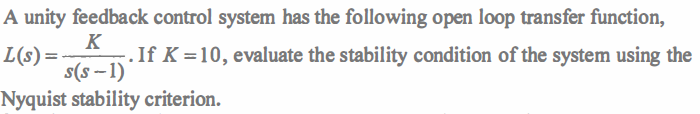A unity feedback control system has the following open loop transfer function, K L(s) .If K 10, evaluate the stability condition of the system using the s(s-1) Nyquist stability criterion. A unity feedback control system has the following open loop transfer function, K L(s) .If K 10, evaluate the stability condition of the system using the s(s-1) Nyquist stability criterion.

• ### Consider a unity feedback control system with open loop transfer function KG(G) s(s+2)(s + 6) 1....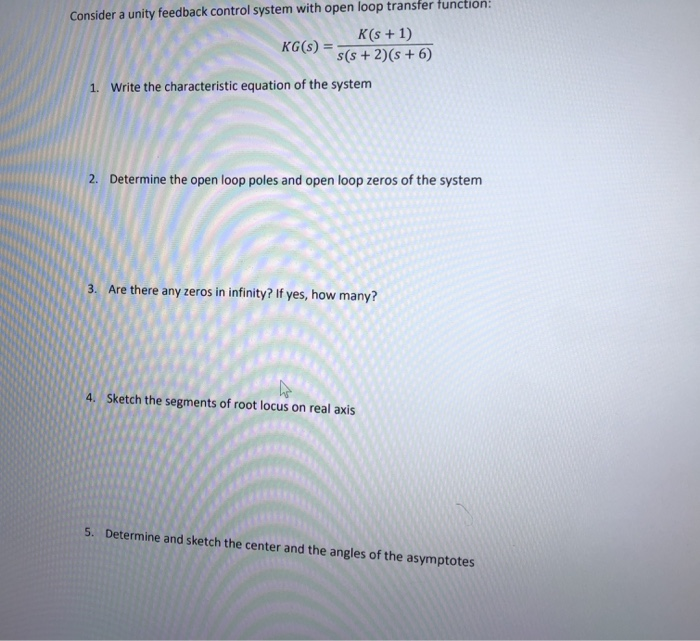Consider a unity feedback control system with open loop transfer function KG(G) s(s+2)(s + 6) 1. Write the characteristic equation of the system 2. Determine the open loop poles and open loop zeros of the system 3. Are there any zeros in infinity? If yes, how many? 4. Sketch the segments of root locus on real axis 5. Determine and sketch the center and the angles of the asymptotes

• ### 2. Consider a unity feedback control system whose open-loop transfer function is K(s-2) G(s) (s+1)(s +6s...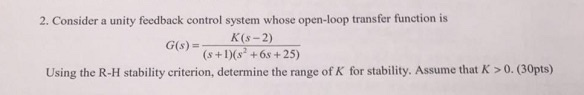2. Consider a unity feedback control system whose open-loop transfer function is K(s-2) G(s) (s+1)(s +6s +25) Using the R-H stability criterion, determine the range of K for stability. Assume that K > 0. (30pts)

• ### Sketch the root locus plot of a unity feedback system with an open loop transfer function...

Sketch the root locus plot of a unity feedback system with an open loop transfer function G(s) = K / s (s+2) (s+4) Determine the value of K so that the dominant pair of complex poles of the system has a damping ratio of 0.5.

• ### 7. Consider a unity feedback control system with open-loop transfer function G(s) = k 5 s...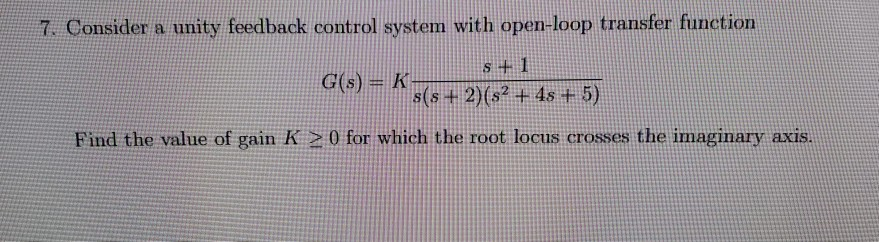7. Consider a unity feedback control system with open-loop transfer function G(s) = k 5 s + 2)(52 + 4s + 5) Find the value of gain K > 0 for which the root locus crosses the imaginary axis.

• ### 4. (10 points) For the feedback system with open loop transfer function 16/3 a. (6 pts) Draw the Root Locus and Nyquist plot of the system with no 16/3 S41 Using Nyquist stability criterion, find...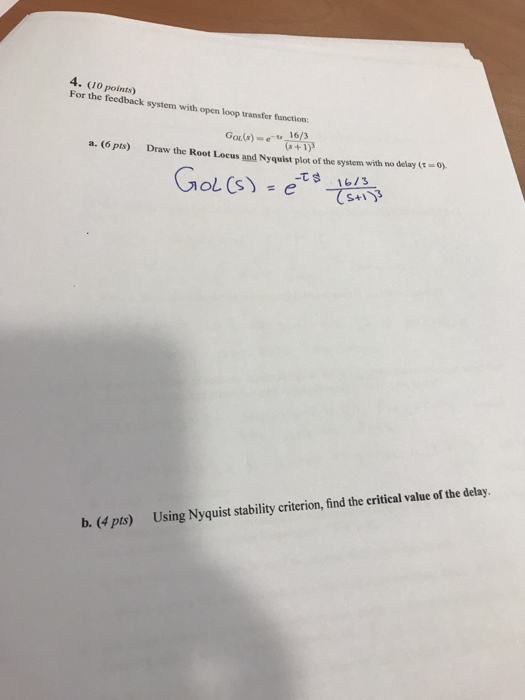4. (10 points) For the feedback system with open loop transfer function 16/3 a. (6 pts) Draw the Root Locus and Nyquist plot of the system with no 16/3 S41 Using Nyquist stability criterion, find the critical value of the delay. b. (4 pts) 4. (10 points) For the feedback system with open loop transfer function 16/3 a. (6 pts) Draw the Root Locus and Nyquist plot of the system with no 16/3 S41 Using Nyquist stability criterion, find the...

• ### Due Date: April 20, 2 Problem 2: Consider a unity-feedback control system with the following open-loop...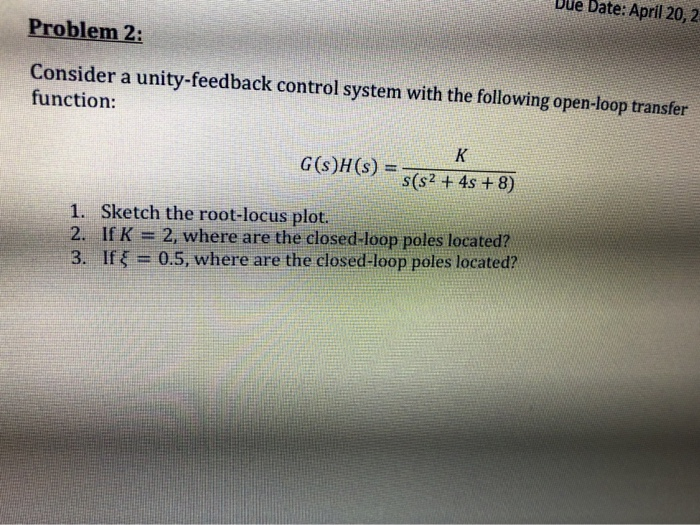Due Date: April 20, 2 Problem 2: Consider a unity-feedback control system with the following open-loop transfer function: K G(s)H(s) = s(s2 + 4s + 8) 1. Sketch the root-locus plot. 2. IfK 2, where are the closed-loop poles located? 3. If x = 0.5, where are the closed-loop poles located?

• ### PD & PID controller design Consider a unity feedback system with open loop transfer function, G(s)...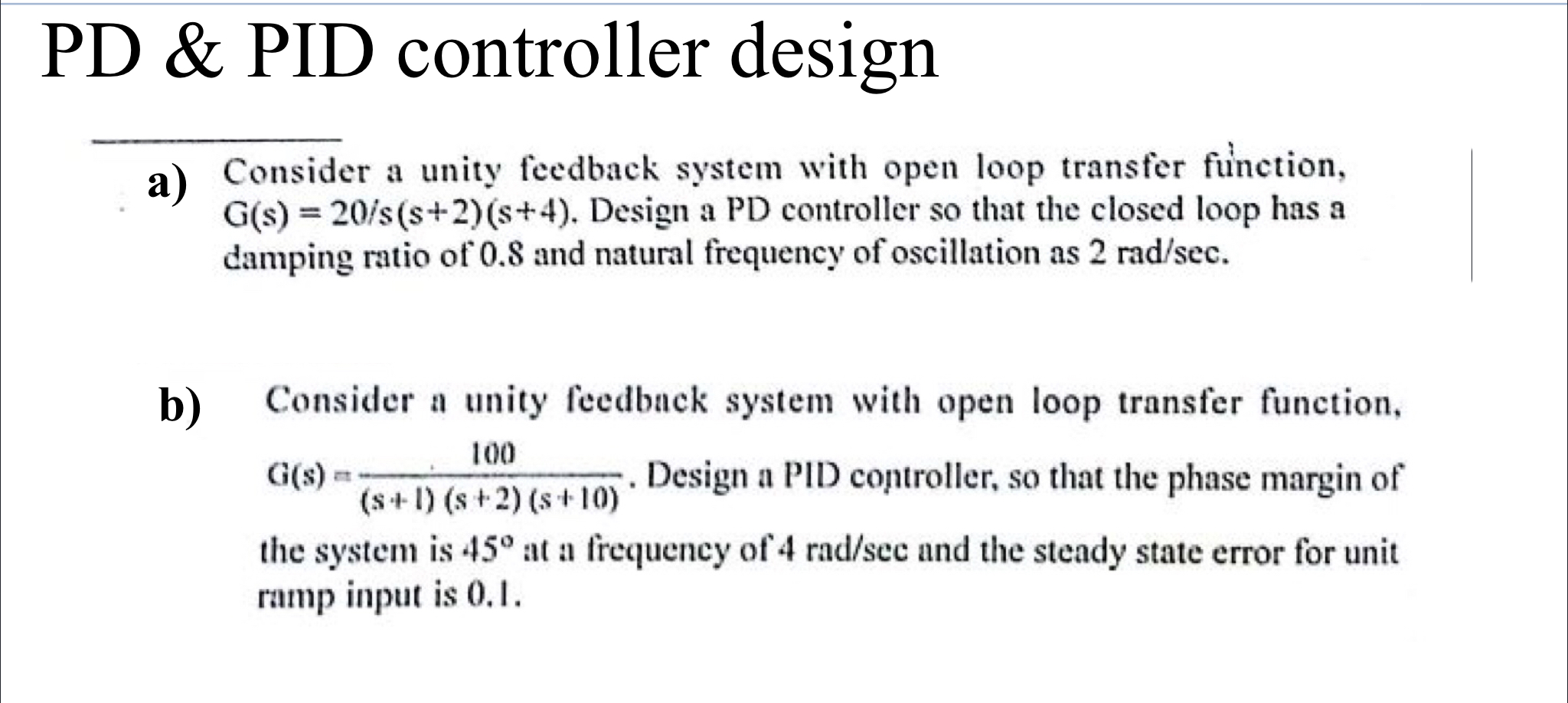PD & PID controller design Consider a unity feedback system with open loop transfer function, G(s) = 20/s(s+2)(8+4). Design a PD controller so that the closed loop has a damping ratio of 0.8 and natural frequency of oscillation as 2 rad/sec. b) 100 Consider a unity feedback system with open loop transfer function, aus. Design a PID controller, so that the phase margin of (S-1) (s + 2) (s+10) the system is 45° at a frequency of 4 rad/scc and...